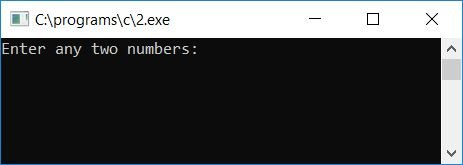# C Program to Find the Sum of Squares of Digits of a Number

In this tutorial, we will learn how to create a program in C that will find and print the sum of squares of any two numbers and also how to find and print the sum of squares of digits of any given number given by the user at run-time.

## C Find the sum of any two numbers' squares

Let's first create a program in C that will ask the user to enter any two numbers to find and print the sum of the square of the given two numbers.

```#include<stdio.h>
#include<conio.h>
int main()
{
int num1, num2, sqr1, sqr2, sum;
printf("Enter any two numbers: ");
scanf("%d%d", &num1, &num2);
sqr1 = num1*num1;
sqr2 = num2*num2;
sum = sqr1 + sqr2;
printf("Sum of their square = %d", sum);
getch();
return 0;
}```

As the program was written in the Code::Blocks IDE, here is the sample run after a successful build and run:Now supply any two numbers, say 4 and 5, and press the ENTER key to see the result, which will be the sum of the square of the given two numbers. If you square two numbers, say 4 and 5, you will get 16 and 25. After summing it up, you will get 16 + 25 = 41 as a result. Here is the second snapshot of the sample run:Here are some of the main steps used in the above program:

• Receive any two numbers as input from the user (at run-time) in any two variables, say num1 and num2.
• Calculate the square of each number one by one and initialize its square to any two variables, say sqr1 and sqr2.
• That is, num1*num1 gets initialized to sqr1, and num2*num2 gets initialized to sqr2.
• Now calculate the sum of two variables, say sqr1 (square of the first number) and sqr2 (square of the second number).
• That is, sqr1 + sqr2 are initialized to a variable called sum (which stores the sum of the squares of the two given numbers).
• At the end of the program, print the sum value.
• For example, if the user enters 4 and 5 as input,
• Therefore, num1 holds 4 and num2 holds 5.
• Now sqr1 is initialized to num1*num1 or 4*4 or 16.
• And num2*num2 or 5*5 or 25 is set to sqr2.
• And sqr1+sqr2 or 16+25 or 41 is set to sum.
• As output, print the sum value, which is 41.

## Find the sum of the squares of a number's digits in C

Now let's create a program that will ask the user to enter any number to find and print the sum of the squares of its digits. That is, if the user enters 342 as input, the program will calculate the sum of the squares of its digits, which is (3*3) + (4*4) + (2*2), or 9+16+4 or 29. Let's take a look at the program:

```#include<stdio.h>
#include<conio.h>
int main()
{
int num, rem, sum=0;
printf("Enter any number: ");
scanf("%d", &num);
while(num>0)
{
rem = num%10;
sum = sum + rem*rem;
num = num/10;
}
printf("\nSum of Square of Digits = %d", sum);
getch();
return 0;
}```

Here is the final snapshot of the sample run:Here are some of the main steps used in the above program:

• Receive any number as input.
• Never forget to start a variable, say sum (which holds the sum of a square of digits), with 0.
• That is declaring and initializing 0 to sum at the start of the program.
• After getting input from the user into a variable, say num, follow the steps given below:
• Create a while loop that runs until the value of num is greater than 0.
• Inside the loop, calculate the digit of that number using the modulus operator (%).
• Using the modulus operator or remainder operator, we will get the last digit of the number.
• Find the square of that digit and sum it up with the sum variable, and then initialize it to the sum variable.
• Then divide the value (num) with 10 to skip or delete the last digit.
• For example, if the user enters 2346 as input,
• Therefore, at the first run of the while loop, as the value of num (2346) is greater than 0, program flow goes inside the loop, and num%10 or 2346%10 or 6  gets initialized to rem. Then sum + rem*rem or 0 + 6*6 or 36 gets initialized to sum. And at last, num/10 or 2346/10 or 234 gets initialized to num.
• And then program flow goes inside the while loop's condition statement, and as the value 234 is again greater than 0, therefore again the condition of the while loop evaluates to true, program flow goes inside the loop, and it does the same steps as told above.
• That is, num%10 or 234%10 or 4 gets initialized to rem. And sum + rem*rem, or 36 + 4*4, or 36 + 16 or 52, gets initialized to sum. Finally, num/10, 234/10, or 23 is initialized to num.
• Again at the third run of the while loop, as the condition statement of the loop, that is, num>0 or 23>0, evaluates to true, the program flow again goes inside the while loop and follows the same procedure until the value of num becomes 0.
• At the last run of the while loop, the variable sum holds its value as 6*6 + 4*4 + 3*3 + 2*2 or 36 + 16 + 9 + 4 or 65 as its final value; therefore, 65 is the final output, which is the result of the sum of the square of digits of the given number, which is 2346.

C Quiz

« Previous Program Next Program »